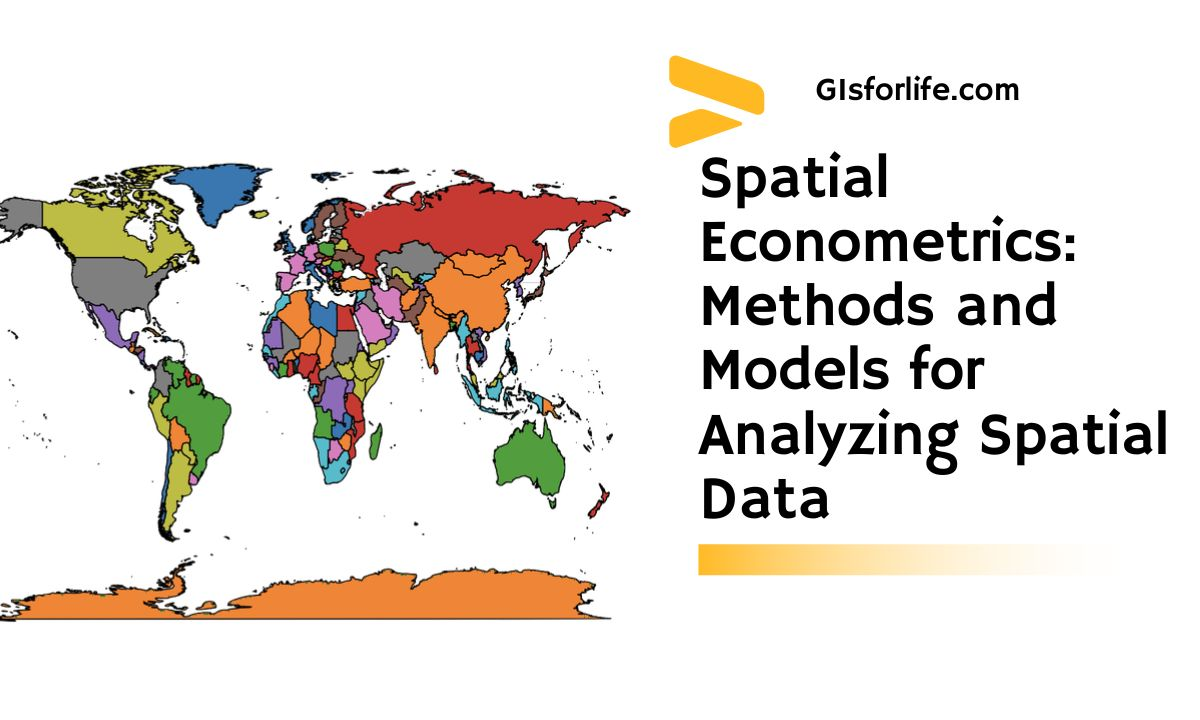## Spatial Econometrics: Methods and Models for Analyzing Spatial Data### Learn about the various methods and models used in spatial econometrics to analyze spatial data

Spatial econometrics is a branch of economics that deals with the spatial aspects of economic phenomena. It studies the relationships between economic variables that are distributed across space. Spatial data refers to data that are associated with a specific location or area. Spatial econometrics methods and models are used to analyze spatial data and explore the spatial relationships between economic variables. This article aims to provide an overview of spatial econometrics methods and models.

### Spatial Data and Spatial Dependence

Spatial data is data that have a specific location or area associated with them. In spatial econometrics, spatial dependence refers to the fact that the values of an economic variable at one location are dependent on the values of the same or related variables at nearby locations. Spatial dependence can arise due to various reasons, such as the diffusion of information, spatially varying economic conditions, and geographical barriers.

Spatial econometric methods are used to account for spatial dependence in spatial data. These methods take into account the spatial relationships between the variables and enable us to make more accurate predictions and estimations.

## Spatial Econometrics Methods

Spatial econometric methods can be broadly classified into two categories: spatial regression models and spatial autoregressive models.

### Spatial Regression Models

Spatial regression models are used to model the relationship between a dependent variable and one or more independent variables that are spatially referenced. The models can be classified into global and local models based on the way spatial dependence is accounted for.

Global models use a single spatial weight matrix to capture the spatial relationships between the variables. Local models, on the other hand, use different weight matrices for different locations to capture the local spatial relationships.

### Spatial Autoregressive Models

Spatial autoregressive models are used to model the dependence between a variable and its neighboring variables. The models assume that the value of a variable at a specific location is a function of the values of the same variable at neighboring locations. Spatial autoregressive models are classified into spatial lag models and spatial error models.

Spatial lag models assume that the value of a variable at a specific location is a function of the values of the same variable at neighboring locations, as well as the values of the other independent variables. Spatial error models, on the other hand, assume that the residuals of the regression model are spatially correlated.

## Spatial Durbin Models

Spatial Durbin models are used to model the simultaneous relationships between a dependent variable, independent variables, and the lagged values of the dependent variable. These models can capture both spatial dependence and endogeneity.

### Spatial Error Models

Spatial error models assume that the residuals of the regression model are spatially correlated. They are used when the dependent variable is not spatially autocorrelated, but the residuals are.

### Geographically Weighted Regression

Geographically weighted regression is a local spatial regression model that uses different weight matrices for different locations to capture the local spatial relationships between the variables. The models enable us to explore the spatial variation in the relationships between the variables.

## Spatial Panel Data Models

Spatial panel data models are used to analyze data that have both spatial and temporal dimensions. These models combine the advantages of spatial econometrics and panel data methods, enabling us to study the spatial and temporal dynamics of economic variables.

## Spatial Analysis of Networks

Spatial analysis of networks is used to study the relationships between economic variables that are connected by a network structure. The models can be used to analyze the diffusion of information, spatially varying economic conditions, and other network-related phenomena.

## FAQs

### What is spatial econometrics?

Spatial econometrics is a branch of economics that deals with the spatial aspects of economic phenomena.

### What is spatial data?

Spatial data is data that have a specific location or area associated with them.

### What is spatial dependence?

Spatial dependence refers to the fact that the values of an economic variable at one location are dependent on the values of the same or related variables at nearby locations.

### What are spatial regression models?

Spatial regression models are used to model the relationship between a dependent variable and one or more independent variables that are spatially referenced.

### What are spatial autoregressive models?

Spatial autoregressive models are used to model the dependence between a variable and its neighboring variables.

### What are spatial panel, data models?

Spatial panel data models are used to analyze data that have both spatial and temporal dimensions.

### What is geographically weighted regression?

Geographically weighted regression is a local spatial regression model that uses different weight matrices for different locations to capture the local spatial relationships between the variables.

### What is the spatial analysis of networks?

Spatial analysis of networks is used to study the relationships between economic variables that are connected by a network structure.

### What are the advantages of spatial econometrics?

Spatial econometrics enables us to analyze spatial data and explore the spatial relationships between economic variables.

### How can spatial econometrics methods be applied in practice?

Spatial econometric methods can be applied in various fields, such as regional economics, urban economics, environmental economics, and transportation economics.

## Conclusion

Spatial econometrics is a powerful tool for analyzing spatial data and exploring the spatial relationships between economic variables. The methods and models discussed in this article can be used to account for spatial dependence, endogeneity, and other spatially related phenomena. Spatial econometrics methods can be applied in various fields, such as regional economics, urban economics, environmental economics, and transportation economics. With the increasing availability of spatial data, spatial econometrics is becoming an increasingly important tool for economists and policymakers.